### Home > CALC > Chapter 1 > Lesson 1.1.1 > Problem1-7

1-7.
1. TRANSLATING FUNCTIONS Homework Help ✎

1. Graph the function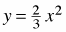. On the same set of axes graph a translation of the function that is shifted one unit to the right and five units down. Write the equation of the translated function.

2. Does the same strategy work for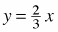? Write an equation that will shiftone unit to the right and five units down.

3. Compare the graphs of and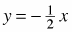and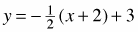. Describe their similarities and differences.

4. Explain how you know that the graph of y = −9(x + 1) − 6 goes through the point (−1, −6) and has a slope of −9.

5. Sketch the graph of y = 5(x − 2) − 1 quickly.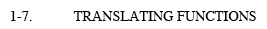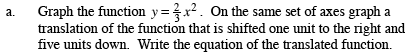$y=\frac{2}{3}(x-1)^2-5$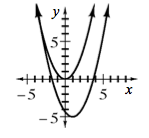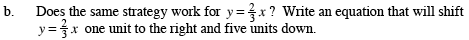$y=\frac{2}{3}(x-k)+v\ \ \ \ \ \ \ \ \text{What are }k\text{ and } v?$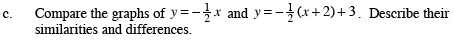Both graphs are straight lines with the same slope. The second graph has been shifted both horizontally and vertically from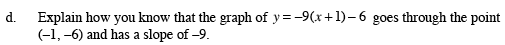This is a straight line in Point-Slope form. See Math Note for more information.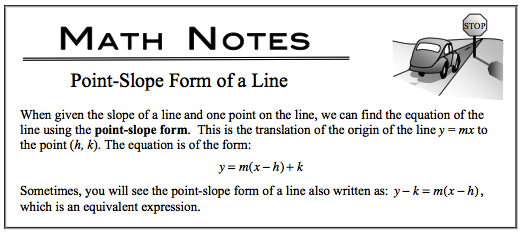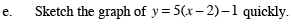Refer to the hint in part (d).